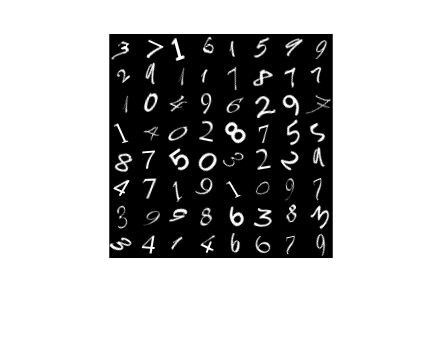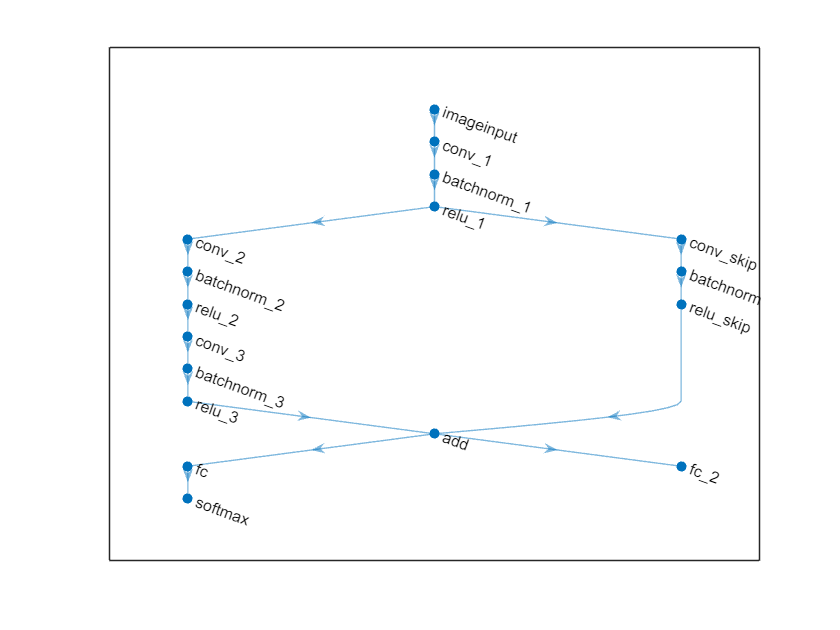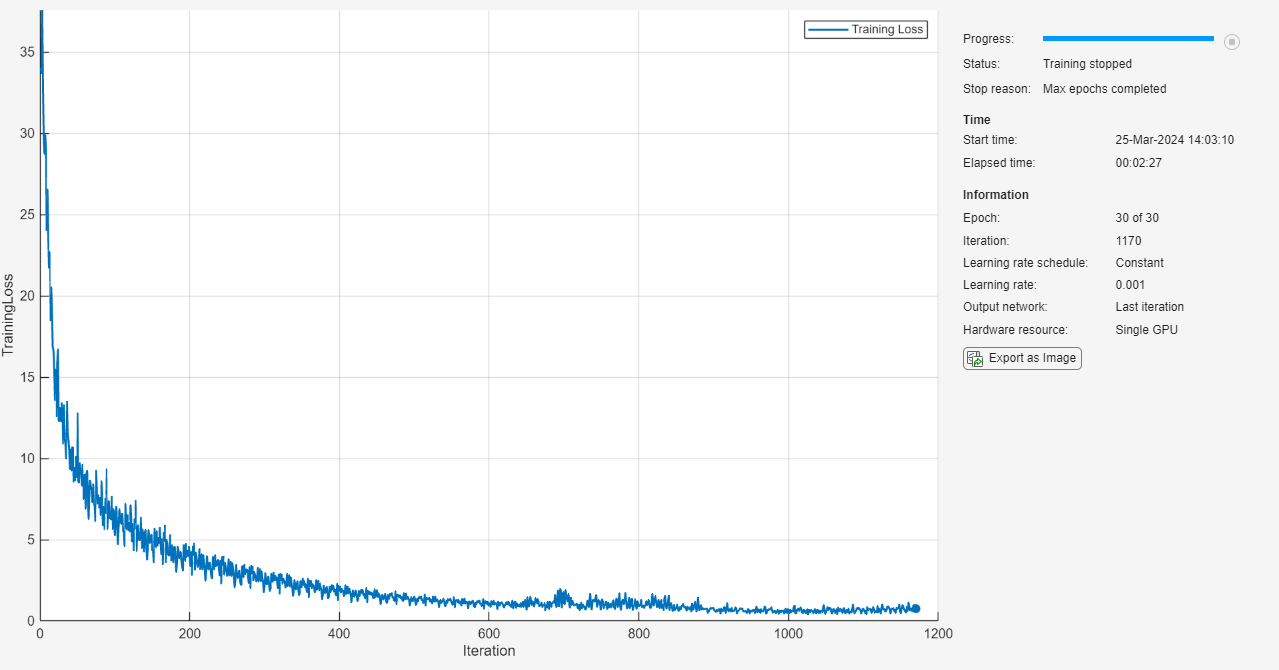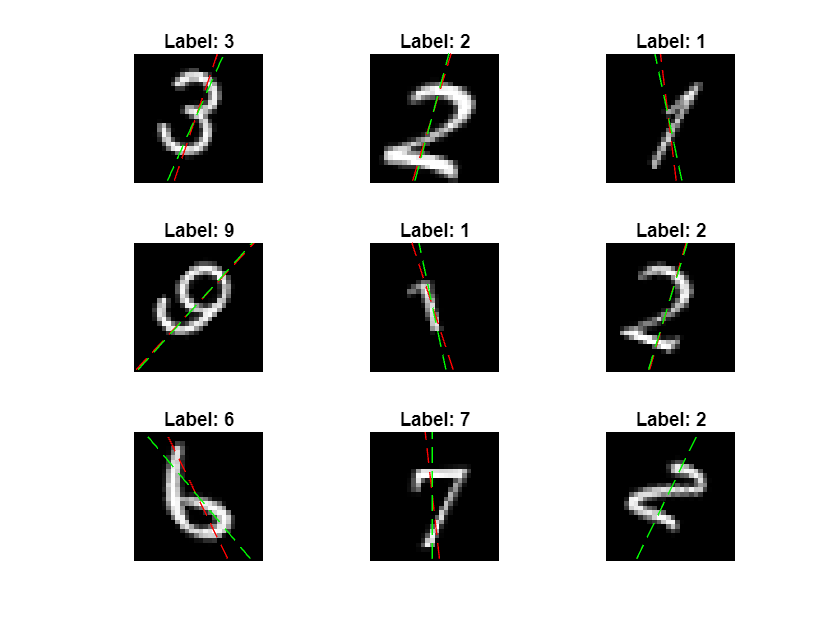Train Network with Multiple Outputs

This example shows how to train a deep learning network with multiple outputs that predict both labels and angles of rotations of handwritten digits.

To train a network with multiple outputs, you must train the network using a custom training loop.

The digitTrain4DArrayData function loads the images, their digit labels, and their angles of rotation from the vertical. Create an arrayDatastore object for the images, labels, and the angles, and then use the combine function to make a single datastore that contains all of the training data. Extract the class names and number of nondiscrete responses.

[XTrain,YTrain,anglesTrain] = digitTrain4DArrayData;

dsXTrain = arrayDatastore(XTrain,'IterationDimension',4);
dsYTrain = arrayDatastore(YTrain);
dsAnglesTrain = arrayDatastore(anglesTrain);

dsTrain = combine(dsXTrain,dsYTrain,dsAnglesTrain);

classNames = categories(YTrain);
numClasses = numel(classNames);
numObservations = numel(YTrain);

View some images from the training data.

idx = randperm(numObservations,64);
I = imtile(XTrain(:,:,:,idx));
figure
imshow(I)Define Deep Learning Model

Define the following network that predicts both labels and angles of rotation.

• A convolution-batchnorm-ReLU block with 16 5-by-5 filters.

• Two convolution-batchnorm-ReLU blocks each with 32 3-by-3 filters.

• A skip connection around the previous two blocks containing a convolution-batchnorm-ReLU block with 32 1-by-1 convolutions.

• Merge the skip connection using addition.

• For classification output, a branch with a fully connected operation of size 10 (the number of classes) and a softmax operation.

• For the regression output, a branch with a fully connected operation of size 1 (the number of responses).

Define the main block of layers as a layer graph.

layers = [
imageInputLayer([28 28 1],'Normalization','none','Name','in')

batchNormalizationLayer('Name','bn1')
reluLayer('Name','relu1')

batchNormalizationLayer('Name','bn2')
reluLayer('Name','relu2')
batchNormalizationLayer('Name','bn3')
reluLayer('Name','relu4')

fullyConnectedLayer(numClasses,'Name','fc1')
softmaxLayer('Name','softmax')];

lgraph = layerGraph(layers);

layers = [
convolution2dLayer(1,32,'Stride',2,'Name','convSkip')
batchNormalizationLayer('Name','bnSkip')
reluLayer('Name','reluSkip')];

lgraph = connectLayers(lgraph,'relu1','convSkip');

Add the fully connected layer for regression.

layers = fullyConnectedLayer(1,'Name','fc2');

View the layer graph in a plot.

figure
plot(lgraph)Create a dlnetwork object from the layer graph.

dlnet = dlnetwork(lgraph)
dlnet =
dlnetwork with properties:

Layers: [17×1 nnet.cnn.layer.Layer]
Connections: [17×2 table]
Learnables: [20×3 table]
State: [8×3 table]
InputNames: {'in'}
OutputNames: {'softmax'  'fc2'}

Create the function modelGradients, listed at the end of the example, that takes as input, the dlnetwork object dlnet, a mini-batch of input data dlX with corresponding targets T1 and T2 containing the labels and angles, respectively, and returns the gradients of the loss with respect to the learnable parameters, the updated network state, and the corresponding loss.

Specify Training Options

Specify the training options. Train for 30 epochs using a mini-batch size of 128.

numEpochs = 30;
miniBatchSize = 128;

Visualize the training progress in a plot.

plots = "training-progress";

Train Model

Use minibatchqueue to process and manage the mini-batches of images. For each mini-batch:

• Use the custom mini-batch preprocessing function preprocessMiniBatch (defined at the end of this example) to one-hot encode the class labels.

• Format the image data with the dimension labels 'SSCB' (spatial, spatial, channel, batch). By default, the minibatchqueue object converts the data to dlarray objects with underlying type single. Do not add a format to the class labels or angles.

• Train on a GPU if one is available. By default, the minibatchqueue object converts each output to a gpuArray if a GPU is available. Using a GPU requires Parallel Computing Toolbox™ and a supported GPU device. For information on supported devices, see GPU Support by Release (Parallel Computing Toolbox).

mbq = minibatchqueue(dsTrain,...
'MiniBatchSize',miniBatchSize,...
'MiniBatchFcn', @preprocessData,...
'MiniBatchFormat',{'SSCB','',''});

Train the model using a custom training loop. For each epoch, shuffle the data and loop over mini-batches of data. At the end of each iteration, display the training progress. For each mini-batch:

• Evaluate the model gradients and loss using dlfeval and the modelGradients function.

• Update the network parameters using the adamupdate function.

Initialize the training progress plot.

if plots == "training-progress"
figure
lineLossTrain = animatedline('Color',[0.85 0.325 0.098]);
ylim([0 inf])
xlabel("Iteration")
ylabel("Loss")
grid on
end

trailingAvg = [];
trailingAvgSq = [];

Train the model.

iteration = 0;
start = tic;

% Loop over epochs.
for epoch = 1:numEpochs

% Shuffle data.
shuffle(mbq)

% Loop over mini-batches
while hasdata(mbq)

iteration = iteration + 1;

[dlX,dlY1,dlY2] = next(mbq);

% Evaluate the model gradients, state, and loss using dlfeval and the
dlnet.State = state;

% Update the network parameters using the Adam optimizer.
trailingAvg,trailingAvgSq,iteration);

% Display the training progress.
if plots == "training-progress"
D = duration(0,0,toc(start),'Format','hh:mm:ss');
title("Epoch: " + epoch + ", Elapsed: " + string(D))
drawnow
end
end
endTest Model

Test the classification accuracy of the model by comparing the predictions on a test set with the true labels and angles. Manage the test data set using a minibatchqueue object with the same setting as the training data.

[XTest,Y1Test,anglesTest] = digitTest4DArrayData;

dsXTest = arrayDatastore(XTest,'IterationDimension',4);
dsYTest = arrayDatastore(Y1Test);
dsAnglesTest = arrayDatastore(anglesTest);

dsTest = combine(dsXTest,dsYTest,dsAnglesTest);

mbqTest = minibatchqueue(dsTest,...
'MiniBatchSize',miniBatchSize,...
'MiniBatchFcn', @preprocessData,...
'MiniBatchFormat',{'SSCB','',''});

To predict the labels and angles of the validation data, loop over the mini-batches and use the predict function. Store the predicted classes and angles. Compare the predicted and true classes and angles and store the results.

classesPredictions = [];
anglesPredictions = [];
classCorr = [];
angleDiff = [];

% Loop over mini-batches.
while hasdata(mbqTest)

[dlXTest,dlY1Test,dlY2Test] = next(mbqTest);

% Make predictions using the predict function.
[dlY1Pred,dlY2Pred] = predict(dlnet,dlXTest,'Outputs',["softmax" "fc2"]);

% Determine predicted classes.
Y1PredBatch = onehotdecode(dlY1Pred,classNames,1);
classesPredictions = [classesPredictions Y1PredBatch];

% Dermine predicted angles
Y2PredBatch = extractdata(dlY2Pred);
anglesPredictions = [anglesPredictions Y2PredBatch];

% Compare predicted and true classes
Y1Test = onehotdecode(dlY1Test,classNames,1);
classCorr = [classCorr Y1PredBatch == Y1Test];

% Compare predicted and true angles
angleDiffBatch = Y2PredBatch - dlY2Test;
angleDiff = [angleDiff extractdata(gather(angleDiffBatch))];

end

Evaluate the classification accuracy.

accuracy = mean(classCorr)
accuracy = 0.9814

Evaluate the regression accuracy.

angleRMSE = sqrt(mean(angleDiff.^2))
angleRMSE = single
7.7431

View some of the images with their predictions. Display the predicted angles in red and the correct labels in green.

idx = randperm(size(XTest,4),9);
figure
for i = 1:9
subplot(3,3,i)
I = XTest(:,:,:,idx(i));
imshow(I)
hold on

sz = size(I,1);
offset = sz/2;

thetaPred = anglesPredictions(idx(i));
plot(offset*[1-tand(thetaPred) 1+tand(thetaPred)],[sz 0],'r--')

thetaValidation = anglesTest(idx(i));
plot(offset*[1-tand(thetaValidation) 1+tand(thetaValidation)],[sz 0],'g--')

hold off
label = string(classesPredictions(idx(i)));
title("Label: " + label)
endThe modelGradients function, takes as input, the dlnetwork object dlnet, a mini-batch of input data dlX with corresponding targets T1 and T2 containing the labels and angles, respectively, and returns the gradients of the loss with respect to the learnable parameters, the updated network state, and the corresponding loss.

[dlY1,dlY2,state] = forward(dlnet,dlX,'Outputs',["softmax" "fc2"]);

lossLabels = crossentropy(dlY1,T1);
lossAngles = mse(dlY2,T2);

loss = lossLabels + 0.1*lossAngles;

end

Mini-Batch Preprocessing Function

The preprocessMiniBatch function preprocesses the data using the following steps:

1. Extract the image data from the incoming cell array and concatenate into a numeric array. Concatenating the image data over the fourth dimension adds a third dimension to each image, to be used as a singleton channel dimension.

2. Extract the label and angle data from the incoming cell arrays and concatenate along the second dimension into a categorical array and a numeric array, respectively.

3. One-hot encode the categorical labels into numeric arrays. Encoding into the first dimension produces an encoded array that matches the shape of the network output.

function [X,Y,angle] = preprocessData(XCell,YCell,angleCell)

% Extract image data from cell and concatenate
X = cat(4,XCell{:});
% Extract label data from cell and concatenate
Y = cat(2,YCell{:});
% Extract angle data from cell and concatenate
angle = cat(2,angleCell{:});

% One-hot encode labels
Y = onehotencode(Y,1);

end